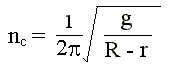### Ball Mill - Operating Speed

In a ball mill of diameter 2000 mm, 100 mm dia steel balls are being used for grinding. Presently, for the material being ground, the mill is run at 15 rpm. At what speed will the mill have to be run if the 100 mm balls are replaced by 50 mm balls, all the other conditions remaining the same?

Calculations:

The critical speed of ball mill is given by,where R = radius of ball mill; r = radius of ball.

For R = 1000 mm and r = 50 mm,

nc = 30.7 rpm

But the mill is operated at a speed of 15 rpm. Therefore, the mill is operated at 100 x 15/30.7 = 48.86 % of critical speed.

If 100 mm dia balls are replaced by 50 mm dia balls, and the other conditions are remaining the same,

Speed of ball mill = [0.4886/(2p)] x [9.812/(1 - 0.025)]0.5

= 14.8 rpm

HOME